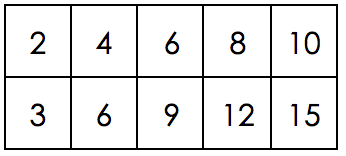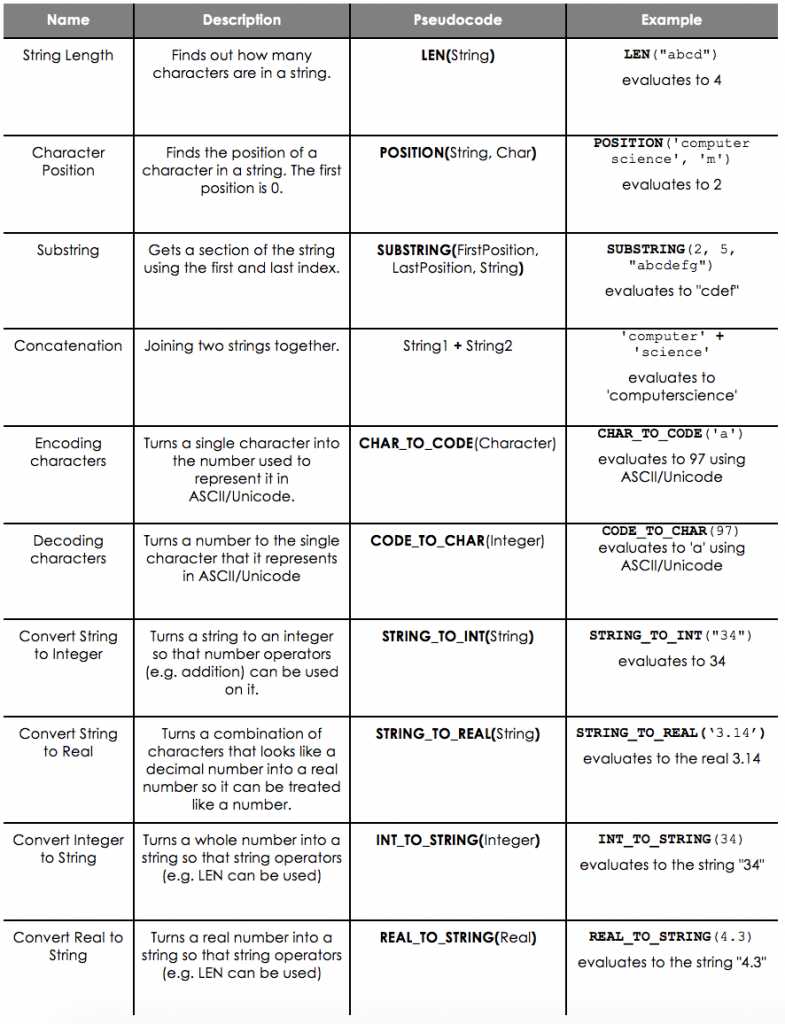# Data Structures

## Arrays

Arrays are lists of values that are grouped together. When we group data items together like this they are treated as one set of data and referred to as a data structure. In an array, all items should be the same data type.One-dimensional Arrays

Here is an example of a 1-dimensional array.

primes ← [ 2, 3, 5, 7, 11, 13 ]

This array has 6 elements consisting of the first 6 primes. We can find out the length of an array using:

LEN(primes)

Having an array like this allows us to store lots of different values in one variable.

Here for example we can get the number 5 using:

primes

The number inside the square brackets shows the index (position) of the value we want to get from the array primes. Notice that the number 5 is in index 2. This is because the first element in an array is in index 0.

We can also use this technique to update values in an array using this

primes ← 17

Array is now [ 2, 3, 5, 7, 11 ,17 ]

Sometimes we want to create something that looks more like a table with multiple rows and columns. We can do this using a 2-dimensional array.

multiples ← [ [ 2, 4, 6, 8, 10 ], [ 3, 6, 9, 12, 15 ] ]

Here we have an array of arrays so we could visualise it like this:If we wanted to get hold of a particular value, we can use a similar technique to the 1-dimensional array. This time, the first value tells us the ‘row’ and the second one tells us the ‘column’.

For example:

multiples == 8, multiples == 15

What are the values of the following:

multiples
2
multiples
4
multiples
10
multiples ==
9
multiples ==
12

## Data Types

When we write programs, the data we use is stored in variables which often have to be declared. The information in each variable can be very different. For example you might have a variable called birth_date which holds a person’s date of birth or you could have a variable called age_of_dog which holds the age of somebody’s dog in years. They both are stored in variables but they have different data types – one is a piece of text and one is an integer (whole number).

When you set up a variable you must decide what data type it is.

But the ones you need to know are:

1. string – any amount of text (letters, numbers and symbols)
2. integer – a whole number
3. real – a number with a part after the decimal point
4. character – one single ‘character’ of a string i.e. a letter, number, symbol
5. boolean – True or False

What is the data type of the following variables?

age = 3
paid = True
boolean
name = 'George'
string
price = 1.50
real
gender = 'M'
phone_number = '01234 560928'
string
Explanation: Notice the quote marks - this means it is a string. If it were stored as an integer, it would lose the 0 at the beginning.

## Strings

A string is any combination of characters. There are lots of things that we can do to strings.## Records

So far we have only looked at the temporary storage of data using variables and arrays. However sometimes data needs to be stored permanently using files. One example of information that might be stored in a file is a group of records.

A record is similar to an array but all of the items don’t need to be the same type. Individual data items within a record are known as fields and instead of accessing the data using the index, we would access it using the field name instead.